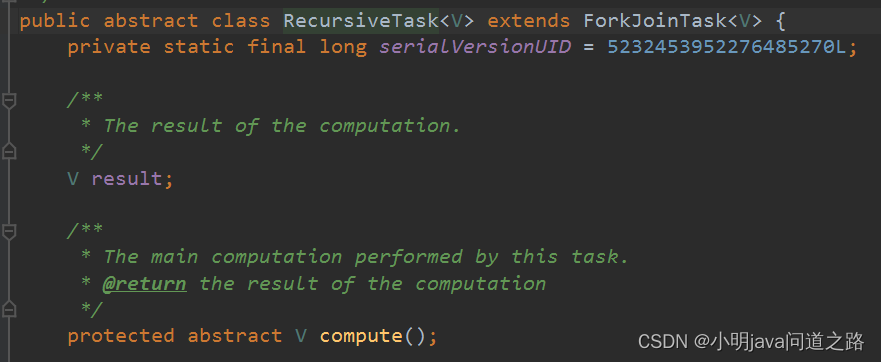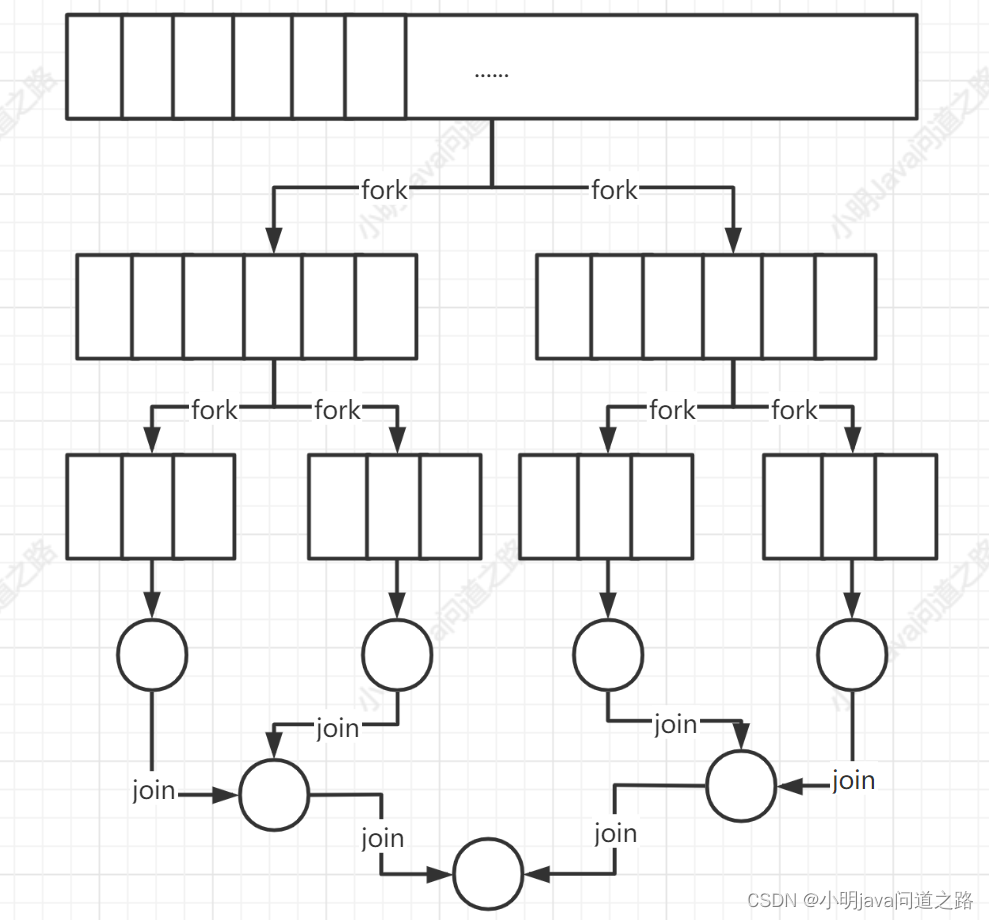> 文档中心 > 【函数式编程实战】(六) Stream高并发实战

# 【函数式编程实战】(六) Stream高并发实战📫 作者简介：小明java问道之路，专注于研究计算机底层，就职于金融公司后端高级工程师，擅长交易领域的高安全/可用/并发/性能的设计和架构📫
🏆 Java领域优质创作者、阿里云专家博主、华为云享专家🏆
🔥 如果此文还不错的话，还请👍关注点赞收藏三连支持👍一下博主哦

# 一、并行流（parallelStream、parallel、sequential）

parallelStream、.parallel()都可以将流转换成并行流，.parallel()的粒度更小。要注意的是顺序流( .stream) 调用parallel方法不意味本身有什么实际变化，它内部设置了一个boolean，表示调用parallel之后的操作都是并行的

`` List collect10 = orderInfos.stream().parallel()  .map(OrderInfo::getOrderId).collect(toList()); BigDecimal totalSubOrderAmt1 = orderInfos.stream()  .map(OrderInfo::getSubOrderInfoList)  .flatMap(subOrderInfos -> subOrderInfos.stream())  .filter(subOrderInfo -> null != subOrderInfo.getSubOrderAmt())  .parallel()  .map(SubOrderInfo::getSubOrderAmt)  .sequential()  .reduce(BigDecimal.ZERO, BigDecimal::add); List collect11 = orderInfos.parallelStream()  .map(OrderInfo::getOrderId).collect(toList());``

# 二、Fork/Jion框架``    public static void main(String[] args) { List orderInfos = Arrays.asList(new OrderInfo("123", BigDecimal.ONE),  new OrderInfo("456", BigDecimal.TEN), new OrderInfo("789", BigDecimal.TEN)); // 创建一个 ForkJoinTask,并把对象传给我们自定义的ForkJoinCalculator ForkJoinCalculator orderInfoForkJoinTask = new ForkJoinCalculator(orderInfos, 0, orderInfos.size()); // 创建一个 ForkJoinPool 并把任务传递给他的调用方法，返回值就是ForkJoinCalculator定义的结果 System.out.println(new ForkJoinPool().invoke(orderInfoForkJoinTask));    }    /**     * 扩展RecursiveTask，穿件ForkJoin框架，创建起始位置和终止位置     */    static class ForkJoinCalculator extends RecursiveTask { List orderInfos; int start; int end; BigDecimal amt; public ForkJoinCalculator(List orderInfos, int start, int end) {     this.orderInfos = orderInfos;     this.amt = orderInfos.get(start).getOrderAmt();     this.start = start;     this.end = end; } @Override protected BigDecimal compute() {     int length = end - start;     // 如果大小等于阈值，返回结果     if (length < 2)  return amt; // 订单金额     ForkJoinCalculator task = new ForkJoinCalculator(orderInfos, start, start + length / 2);     task.fork();     ForkJoinCalculator task1 = new ForkJoinCalculator(orderInfos, start + length / 2, end);     BigDecimal compute = task1.compute();     BigDecimal join = task.join();     System.out.println("compute:" + compute + "  join:" + join);     return compute.add(join); // 累加 }    }``